# Napoleon points

In geometry, Napoleon points are a pair of special points associated with a plane triangle. It is generally believed that the existence of these points was discovered by Napoleon Bonaparte, the Emperor of the French from 1804 to 1815, but many have questioned this belief. The Napoleon points are triangle centers and they are listed as the points X(17) and X(18) in Clark Kimberling's Encyclopedia of Triangle Centers.

The name "Napoleon points" has also been applied to a different pair of triangle centers, better known as the isodynamic points.

## Definition of the points

### First Napoleon point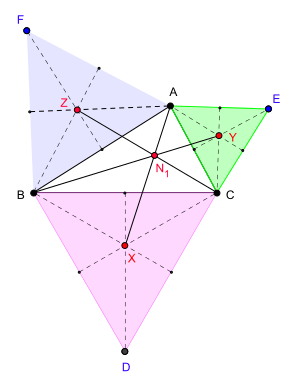First Napoleon point

Let ABC be any given plane triangle. On the sides BC, CA, AB of the triangle, construct outwardly drawn equilateral triangles DBC, ECA and FAB respectively. Let the centroids of these triangles be X, Y and Z respectively. Then the lines AX, BY and CZ are concurrent. The point of concurrence N1 is the first Napoleon point, or the outer Napoleon point, of the triangle ABC.

The triangle XYZ is called the outer Napoleon triangle of the triangle ABC. Napoleon's theorem asserts that this triangle is an equilateral triangle.

In Clark Kimberling's Encyclopedia of Triangle Centers, the first Napoleon point is denoted by X(17).### Second Napoleon point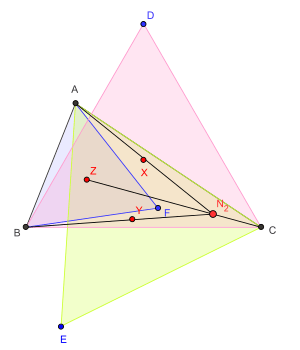Second Napoleon point

Let ABC be any given plane triangle. On the sides BC, CA, AB of the triangle, construct inwardly drawn equilateral triangles DBC, ECA and FAB respectively. Let the centroids of these triangles be X, Y and Z respectively. Then the lines AX, BY and CZ are concurrent. The point of concurrence N2 is the second Napoleon point, or the inner Napoleon point, of the triangle ABC.

The triangle XYZ is called the inner Napoleon triangle of the triangle ABC. Napoleon's theorem asserts that this triangle is an equilateral triangle.

In Clark Kimberling's Encyclopedia of Triangle Centers, the second Napoleon point is denoted by X(18).

• The trilinear coordinates of N2:• The barycentric coordinates of N2:Two points closely related to the Napoleon points are the Fermat-Torricelli points (ETC's X13 and X14). If instead of constructing lines joining the equilateral triangles' centroids to the respective vertices one now constructs lines joining the equilateral triangles' apices to the respective vertices of the triangle, the three lines so constructed are again concurrent. The points of concurrence are called the Fermat-Torricelli points, sometimes denoted F1 and F2. The intersection of the Fermat line (i.e., that line joining the two Fermat-Torricelli points) and the Napoleon line (i.e., that line joining the two Napoleon points) is the triangle's symmedian point (ETC's X6).

## Generalizations

The results regarding the existence of the Napoleon points can be generalized in different ways. In defining the Napoleon points we begin with equilateral triangles drawn on the sides of the triangle ABC and then consider the centers X, Y, and Z of these triangles. These centers can be thought as the vertices of isosceles triangles erected on the sides of triangle ABC with the base angles equal to π/6 (30 degrees). The generalizations seek to determine other triangles that, when erected over the sides of the triangle ABC, have concurrent lines joining their external vertices and the vertices of triangle ABC.

### Isosceles triangles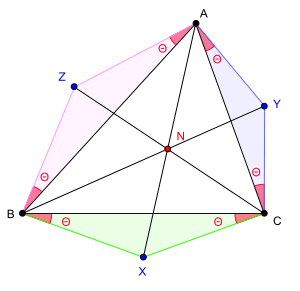A point on the Kiepert hyperbola.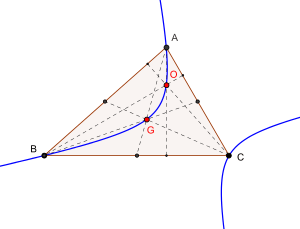Kiepert hyperbola of triangle ABC. The hyperbola passes through the vertices (A,B,C), the orthocenter (O) and the centroid (G) of the triangle.

This generalization asserts the following:

If the three triangles XBC, YCA and ZAB, constructed on the sides of the given triangle ABC as bases, are similar, isosceles and similarly situated, then the lines AX, BY, CZ concur at a point N.

If the common base angle is, then the vertices of the three triangles have the following trilinear coordinates.

•••The trilinear coordinates of N areA few special cases are interesting.

Value of θ; The point N
0 G, the centroid of triangle ABC
π/2 (or –π/2) O, the orthocenter of triangle ABC
π/4 (or –π/4) The Vecten points
π/6 N1, the first Napoleon point (X17)
π/6 N2, the second Napoleon point (X18)
π/3 F1, the first Fermat–Torricelli point (X13)
π/3 F2, the second Fermat–Torricelli point (X14)
A (if A < π/2)
πA (if A > π/2)
The vertex A
B (if B < π/2)
πB (if B > π/2)
The vertex B
C (if C < π/2)
πC (if C > π/2)
The vertex C

Moreover, the locus of N as the base anglevaries between −π/2 and π/2 is the conicThis conic is a rectangular hyperbola and it is called the Kiepert hyperbola in honor of Ludwig Kiepert (1846–1934), the mathematician who discovered this result. This hyperbola is the unique conic which passes through the five points A, B, C, G and O.

### Similar triangles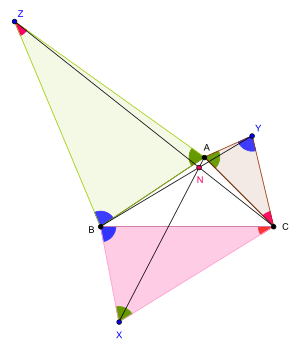Generalization of Napoleon point: A special case

The three triangles XBC, YCA, ZAB erected over the sides of the triangle ABC need not be isosceles for the three lines AX, BY, CZ to be concurrent.

If similar triangles XBC, AYC, ABZ are constructed outwardly on the sides of any triangle ABC then the lines AX, BY and CZ are concurrent.

### Arbitrary triangles

The concurrence of the lines AX, BY, and CZ holds even in much relaxed conditions. The following result states one of the most general conditions for the lines AX, BY, CZ to be concurrent.

If triangles XBC, YCA, ZAB are constructed outwardly on the sides of any triangle ABC such that
∠CBX = ∠ABZ, ∠ACY = ∠BCX, ∠BAZ = ∠CAY,
then the lines AX, BY and CZ are concurrent.

The point of concurrency is known as the Jacobi point.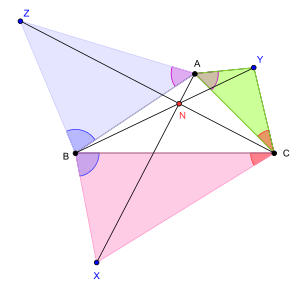A generalization of Napoleon point

## History

Coxeter and Greitzer state the Napoleon Theorem thus: If equilateral triangles are erected externally on the sides of any triangle, their centers form an equilateral triangle. They observe that Napoleon Bonaparte was a bit of a mathematician with a great interest in geometry. However, they doubt whether Napoleon knew enough geometry to discover the theorem attributed to him.

The earliest recorded appearance of the result embodied in Napoleon's theorem is in an article in The Ladies' Diary appeared in 1825. The Ladies' Diary was an annual periodical which was in circulation in London from 1704 to 1841. The result appeared as part of a question posed by W. Rutherford, Woodburn.

VII. Quest.(1439); by Mr. W. Rutherford, Woodburn." Describe equilateral triangles (the vertices being either all outward or all inward) upon the three sides of any triangle ABC: then the lines which join the centers of gravity of those three equilateral triangles will constitute an equilateral triangle. Required a demonstration."

However, there is no reference to the existence of the so-called Napoleon points in this question. Christoph J. Scriba, a German historian of mathematics, has studied the problem of attributing the Napoleon points to Napoleon in a paper in Historia Mathematica.